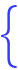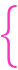Mathematics

Equations
Fold
Math OP
Unfold
On line solution of multivariate equations：
First set the elements of the equation (i.e. the number of unknowns), then click the "Next" button to enter the coefficients of each element of the equation set, and click the "Next" button to obtain the solution of the equation set.
Note that the coefficients of each element of the equation system can only be numbers, not algebraic expressions (including mathematical functions).
Current location：Equations > Multivariate equations > Answer
detailed information：
The input equation set is:15 x -2 y = 3 (1)
 2 x + 3 y = 5 (2)
Question solving process:

Multiply both sides of equation (1) by 2
Divide the two sides of equation (1) by 15, the equation can be obtained:
 2 x - 415 y = 25 (3)
, then subtract both sides of equation (3) from both sides of equation (2), the equations are reduced to:15 x -2 y = 3 (1)
 4915 y = 235 (2)

Multiply both sides of equation (2) by 30
Divide both sides of equation (2) by 49, get the equation：
 2 y = 13849 (4)
, then add the two sides of equation (4) to both sides of equation (1), get the equation：15 x = 28549 (1)
 4915 y = 235 (2)

The coefficient of the unknown number is reduced to 1, and the equations are reduced to：x = 1949 (1)
 y = 6949 (2)

Therefore, the solution of the equation set is:x = 1949
 y = 6949

Convert the solution of the equation set to decimals:x = 0.387755
 y = 1.40816

New addition:Lenders ToolBox module（Specific location：Math OP > Lenders ToolBox ），welcome。